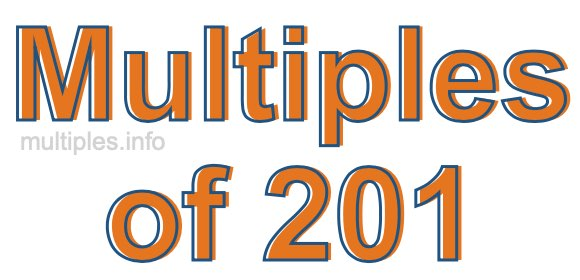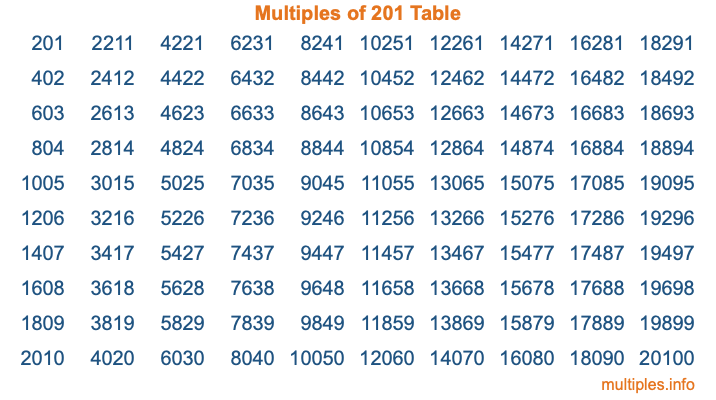Multiples of 201Welcome to the Multiples of 201 page. Here we will first teach you everything you will ever need to know about the multiples of 201, and then give you a study guide summary of everything we taught you to make sure you remember it all. Use this page to look up facts and learn information about the multiples of 201. This page will make you a multiples of two hundred one expert!

Definition of Multiples of 201
Multiples of 201 are all the numbers that when divided by 201 equal an integer. Each of the multiples of 201 are called a multiple. A multiple of 201 is created by multiplying 201 by an integer.

Therefore, to create a list of multiples of 201, you start with 1 multiplied by 201, then 2 multiplied by 201, then 3 multiplied by 201, and so on for as long as you want. Thus, the list of the first five multiples of 201 is 201, 402, 603, 804, and 1005. To see a larger list of multiples of 201, see the printable image of Multiples of 201 further down on this page. We also have a category where you can choose any nth multiple of 201.

Multiples of 201 Checker
The Multiples of 201 Checker below checks to see if any number of your choice is a multiple of 201. In other words, it checks to see if there is any number (integer) that when multiplied by 201 will equal your number. To do that, we divide your number by 201. If the the quotient is an integer, then your number is a multiple of 201.

Is  a multiple of 201?

Least Common Multiple of 201 and ...
A Least Common Multiple (LCM) is the lowest multiple that two or more numbers have in common. This is also called the smallest common multiple or lowest common multiple and is useful to know when you are adding our subtracting fractions. Enter one or more numbers below (201 is already entered) to find the LCM.

Check out our LCM Calculator if you need more details about the Least Common Multiple or if you need the LCM for different numbers for adding and subtraction fractions.

nth Multiple of 201
As we stated above, 201 is the first multiple of 201, 402 is the second multiple of 201, 603 is the third multiple of 201, and so on. Enter a number below to find the nth multiple of 201.

th multiple of 201

Multiples of 201 vs Factors of 201
201 is a multiple of 201 and a factor of 201, but that is where the similarities end. All postive multiples of 201 are 201 or greater than 201. All positive factors of 201 are 201 or less than 201.

Below is the beginning list of multiples of 201 and the factors of 201 so you can compare:

Multiples of 201: 201, 402, 603, 804, 1005, etc.

Factors of 201: 1, 3, 67, 201

As you can see, the multiples of 201 are all the numbers that you can divide by 201 to get a whole number. The factors of 201, on the other hand, are all the whole numbers that you can multiply by another whole number to get 201.

It's also interesting to note that if a number (x) is a factor of 201, then 201 will also be a multiple of that number (x).

Multiples of 201 vs Divisors of 201
The divisors of 201 are all the integers that 201 can be divided by evenly. Below is a list of the divisors of 201.

Divisors of 201: 1, 3, 67, 201

The interesting thing to note here is that if you take any multiple of 201 and divide it by a divisor of 201, you will see that the quotient is an integer.

Multiples of 201 Table
Below is an image of the first 100 multiples of 201 in a table. The table is in chronological order, column by column. The first column has the first ten multiples of 201, the second column has the next ten multiples of 201, and so on.The Multiples of 201 Table is also referred to as the 201 Times Table or Times Table of 201. You are welcome to print out our table for your studies.

Negative Multiples of 201
Although not often discussed or needed in math, it is worth mentioning that you can make a list of negative multiples of 201 by multiplying 201 by -1, then by -2, then by -3, and so on, to get the following list of negative multiples of 201:

-201, -402, -603, -804, -1005, etc.

Multiples of 201 Summary
Below is a summary of important Multiples of 201 facts that we have discussed on this page. To retain the knowledge on this page, we recommend that you read through the summary and explain to yourself or a study partner why they hold true.

There are an infinite number of multiples of 201.

A multiple of 201 divided by 201 will equal a whole number.

201 divided by a factor of 201 equals a divisor of 201.

The nth multiple of 201 is n times 201.

The largest factor of 201 is equal to the first positive multiple of 201.

201 is a multiple of every factor of 201.

201 is a multiple of 201.

A multiple of 201 divided by a divisor of 201 equals an integer.

201 divided by a divisor of 201 equals a factor of 201.

Any integer times 201 will equal a multiple of 201.

Multiples of a Number
Here you can get the multiples of another number, all with the same attention to detail as we did for multiples of 201 on this page.

Multiples of
Multiples of 202
Did you find our page about multiples of two hundred one educational? Do you want more knowledge? Check out the multiples of the next number on our list!